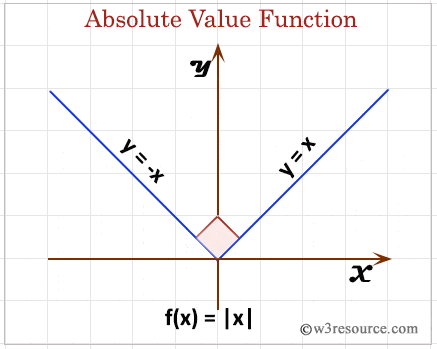# SQL ABS() function

## Absolute value function

SQL ABS() function is used to get the absolute value of a number passed as an argument.

Syntax:

```ABS(expression)
```

Parameters:

Name Description
expression An expression which is a numeric value or numeric data type. The bit data type is not allowed.

MySQL, PostgreSQL, Oracle and SQL Server

All of above platforms support the SQL syntax of ABS().

## SQL ABS() function: Pictorial presentationExample :

To get the absolute value of the number -17.36 from the DUAL table, the following SQL statement can be used :

``````SELECT ABS(-17.36)
FROM dual;
``````

Output:

```ABS(-17.36)
-----------
17.36
```

## SQL ABS() function with distinct clause

Sample table: agents

To get unique absolute value of the column 'commission' after multiplying by (-1) with a column alias "DISTINCT(ABS())" from the 'agents' table, the following SQL statement can be used :

``````SELECT DISTINCT(ABS(commission*(-1))) "DISTINCT(ABS())"
FROM agents;
``````

Output:

```DISTINCT(ABS())
---------------
.15
.11
.14
.13
.12
```

Note: Outputs of the said SQL statement shown here is taken by using Oracle Database 10g Express Edition.

Here is a slide presentation which covers the SQL arithmetic functions.

Practice SQL Exercises